# KSEEB Solutions for Class 9 Maths Chapter 12 Circles Ex 12.4

In this chapter, we provide KSEEB SSLC Class 9 Maths Chapter 12 Circles Ex 12.4 for English medium students, Which will very helpful for every student in their exams. Students can download the latest KSEEB SSLC Class 9 Maths Chapter 12 Circles Ex 12.4 pdf, free KSEEB SSLC Class 9 Maths Chapter 12 Circles Ex 12.4 pdf download. Now you will get step by step solution to each question.

## Karnataka Board Class 9 Maths Chapter 12 Circles Ex 12.4

Question 1.
Two circles of radii 5 cm and 3 cm intersect at two points and the distance between their centres is 4 cm. Find the length of the common chord.
Solution:
R = 5 cm, r = 3 cm. d = 4 cm.
length of the common chord = ?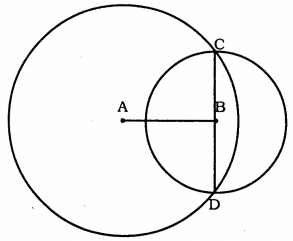When measured Chord CD = 6 cm.
Circles with centre A and B, intersect at points C and D.
CD is common chord.
Perpendicular bisector of CD is AB.
AC = 5cm. BC = 3cm.
∴ Chord, CD= 2 × OC = 2 × 3
∴ Chord, CD = 6 cm.

Question 2.
If two equal chords of a circle intersect within the circle, prove that the segments of one chord are equal to corresponding segments of the other chord.
Solution:
Data : Circle with centre ‘O’, AB and CD chords are equal and intersect at E.
To Prove: Segment EAD = Segment ACB.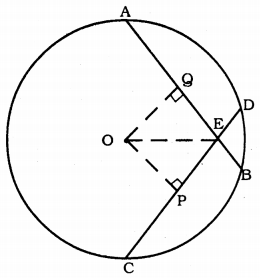Construction: Draw OP⊥CD and OQ⊥AB.
Join OE.
Proof: AQ = QB (∵ Chord drawn perpendicular CP = PD ,to centre bisects chord.).
In ∆OPE and ∆OQE,
∠OPE = ∠OQE = 90° (Construction)
OP = OQ
OE is common.
∴ ∆OPE ≅ ∆OQE (RHS Theorem)
QE = PE ……….. (i)
But QB = PD
∴ QB – QE = PD – PE
EB = ED ……….. (ii)
From (i) and (ii),
AE = CE.
CBD is minor segment formed by Chord CD.
ADB is minor segment formed by Chord AB.
∴ Minor segment CBD = Minor segment ADB
Major segment CAD = Major segment ACB.

Question 3.
If two equal chords of a circle intersect within the circle, prove that the line joining the point of intersection to the centre makes equal angles with the chords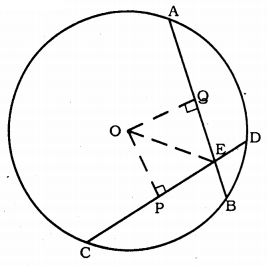Solution:
Data: O is the centre of Circle. Chord AB = Chord CD. These chords intersects at E. OE is joined.
To Prove: ∠OEP = ∠OEQ Construction:
Draw OP⊥CD and OQ⊥AB.
Proof: In ∆OPE and ∆OQE,
∠OPE = ∠OQE = 90° (construction)
OE is common.
OP = OQ (Equal chords are equidistant)
∴ ∆OPE ≅ ∆OEQ (RHS postulate)
∴ ∠OEP = ∠OEQ.

Question 4.
If a line intersects two concentric circles (circles with the same centre) with centre O at A, B, C and D, prove that AB = CD.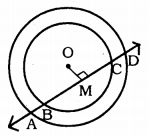Solution:
Data: A line intersects two concentric circles with centre O at A, B, C, and D.
To Prove: AB = CD.
Proof: AD is the chord of a bigger circle.
∴ AM = MD ………(i) (∵ Perpendicular bisects the chord from centre)
Similarly, BC is the chord of the smaller circle.
OM⊥BC.
∴ BM = MC ………. (ii)
Subtracting (ii) from (i),
AM – BM = MD – MC
∴ AB = CD.

Question 5.
Three girls Reshma, Salma and Mandip are playing a game by standing on a circle of radius 5m drawn in a park. Reshma throws a ball to Salma, Salma to Mandip, Mandip to Reshma. If the distance between Reshma and Salma and between Salma and Mandip is 6 m each, what is the distance between Reshma and Mandip?
Solution:
Data: Three girls Reshma, Salma and Mandip are playing a game by standing on a circle of radius 5 cm drawn in a park. Distance between Reshma and Salma and between Salma and Mandip is 6 cm. each.
To Prove: Distance between Reshma and Mandip = ?, RM = ?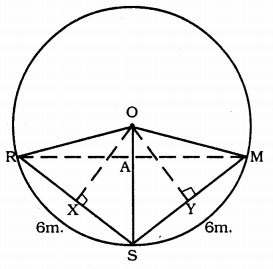Construction: Draw OX⊥RS and OY⊥SM. OR, OS and OM are joined.
Proof: OR = OS = OM = 5 m.
RX = XS = 3 m.
Now, in ⊥∆ORX,
OR2 = OX2 + RX2
(5)2 = (OX)2 + (3)2
25 = OX2 + 9
25 – 9 = OX2
16 = OX2
OX2 = 16
∴ OX = 4 WI …………… (i)
OR = OM and SR = SM
∴ ORSM is a kite.
Diagonals of a kite bisect at the right angle.
∠RAS = 90°
∴ RA = AM.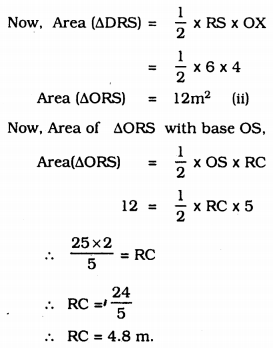Now, RM = 2RC = 2 × 4.8 = 9.8 m
∴ RM = 9.8 m.
∴ Distance between Reshma and Mandip is 9.8 m.

Question 6.
A circular park of radius 20 m is situated in a colony. Three boys Ankur, Syed and David are sitting at equal distance on its boundary each having a toy telephone in his hands to talk each other. Find the length of the string of each phone.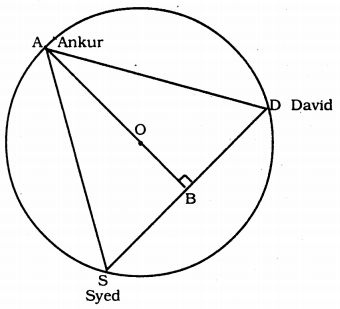Solution:
Data: A circular park of radius 20 m is situated in a colony. Three boys Ankur, Syed, and David are sitting at equal distance on its boundary each having a toy telephone in his hands to talk to each other.
To Prove: Length of wire of telephone =?
Proof: In this figure, AS = SD = DA
∴ ASD is an equilateral triangle.
∴ OA = 20 m. (∵ radius)
Median passed through centre of circle.
∴ AB is the median.
Median of an equilateral triangle divides in the ratio 2 : 1 in the centre?
∴ AO : OB = 2 : 1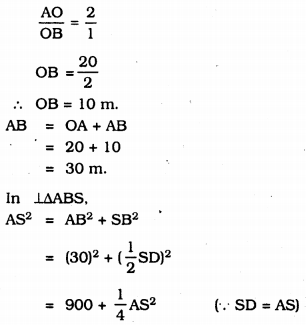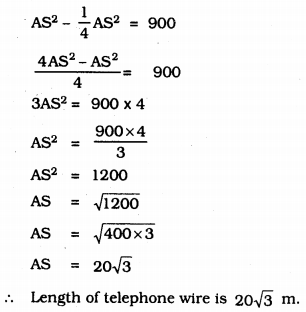All Chapter KSEEB Solutions For Class 9 Maths

—————————————————————————–

All Subject KSEEB Solutions For Class 9

*************************************************

I think you got complete solutions for this chapter. If You have any queries regarding this chapter, please comment on the below section our subject teacher will answer you. We tried our best to give complete solutions so you got good marks in your exam.

If these solutions have helped you, you can also share kseebsolutionsfor.com to your friends.

Best of Luck!!